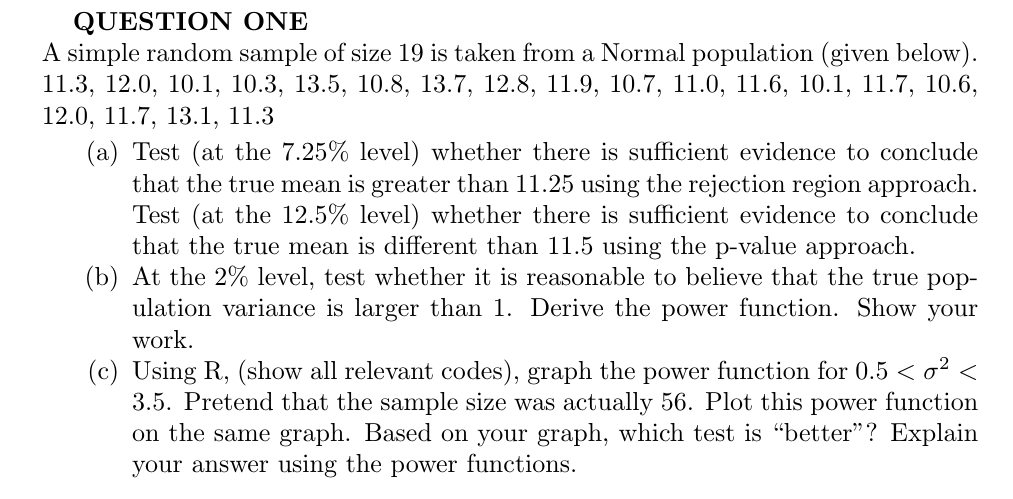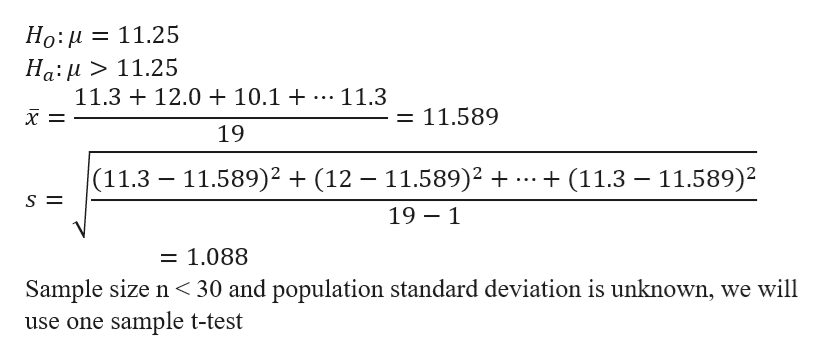# QUESTION ONEA simple random sample of size 19 is taken froma Normal population (given below)11.3, 12.0, 10.1, 10.3, 13.5, 10.8, 13.7, 12.8, 11.9, 10.7, 11.0, 11.6, 10.1, 11.7, 10.6,12.0, 11.7, 13.1, 11.3(a) Test (at the 7.25% level) whether there is sufficient evidence to concludethat the true mean is greater than 11.25 using the rejection region approachTest (at the 12.5% level) whether there is sufficient evidence to concludethat the true mean is different than 11.5 using the p-value approach(b) At the 2% level, test whether it is reasonable to believe that the true population variance is larger than 1. Derive the power function. Show yourwork(c) Using R, (show all relevant codes), graph the power function for 0.5 < o2 <3.5. Pretend that the sample size wason the same graph. Based on your graph, which test is "better"? Explainactually 56. Plot this power functionn

Question
75 views

Please, I need a detailed and a step by step solutions to the problem. I don't have a strong background in statistics and I will appreciate it if you could help out. PLEASE DONT FORGET TO ADD ME THE R CODE FOR SUBQUESTION C. Thank you.help_outlineImage TranscriptioncloseQUESTION ONE A simple random sample of size 19 is taken froma Normal population (given below) 11.3, 12.0, 10.1, 10.3, 13.5, 10.8, 13.7, 12.8, 11.9, 10.7, 11.0, 11.6, 10.1, 11.7, 10.6, 12.0, 11.7, 13.1, 11.3 (a) Test (at the 7.25% level) whether there is sufficient evidence to conclude that the true mean is greater than 11.25 using the rejection region approach Test (at the 12.5% level) whether there is sufficient evidence to conclude that the true mean is different than 11.5 using the p-value approach (b) At the 2% level, test whether it is reasonable to believe that the true pop ulation variance is larger than 1. Derive the power function. Show your work (c) Using R, (show all relevant codes), graph the power function for 0.5 < o2 < 3.5. Pretend that the sample size was on the same graph. Based on your graph, which test is "better"? Explain actually 56. Plot this power function n fullscreen
check_circle

Step 1

Hello, thank you for the question, Part c requires the use of other platform and the solution is too complex for this platform to support it, at the moment. So please find the solution of part a and part b

Step 2

The given data are as follows

Step 3

a) i) Formulation of hypoth...help_outlineImage TranscriptioncloseНо: и 3D 11.25 На:и> 11.25 11.3 12.0 + 10.1 + 11.3 11.589 хE 19 11.589)2(12 - 11.589)2 (11.3 (11.3 11.589)2 19 1 1.088 Sample size n 30 and population standard deviation is unknown, we will use one sample t-test fullscreen

### Want to see the full answer?

See Solution

#### Want to see this answer and more?

Solutions are written by subject experts who are available 24/7. Questions are typically answered within 1 hour.*

See Solution
*Response times may vary by subject and question.
Tagged in

### Statistics